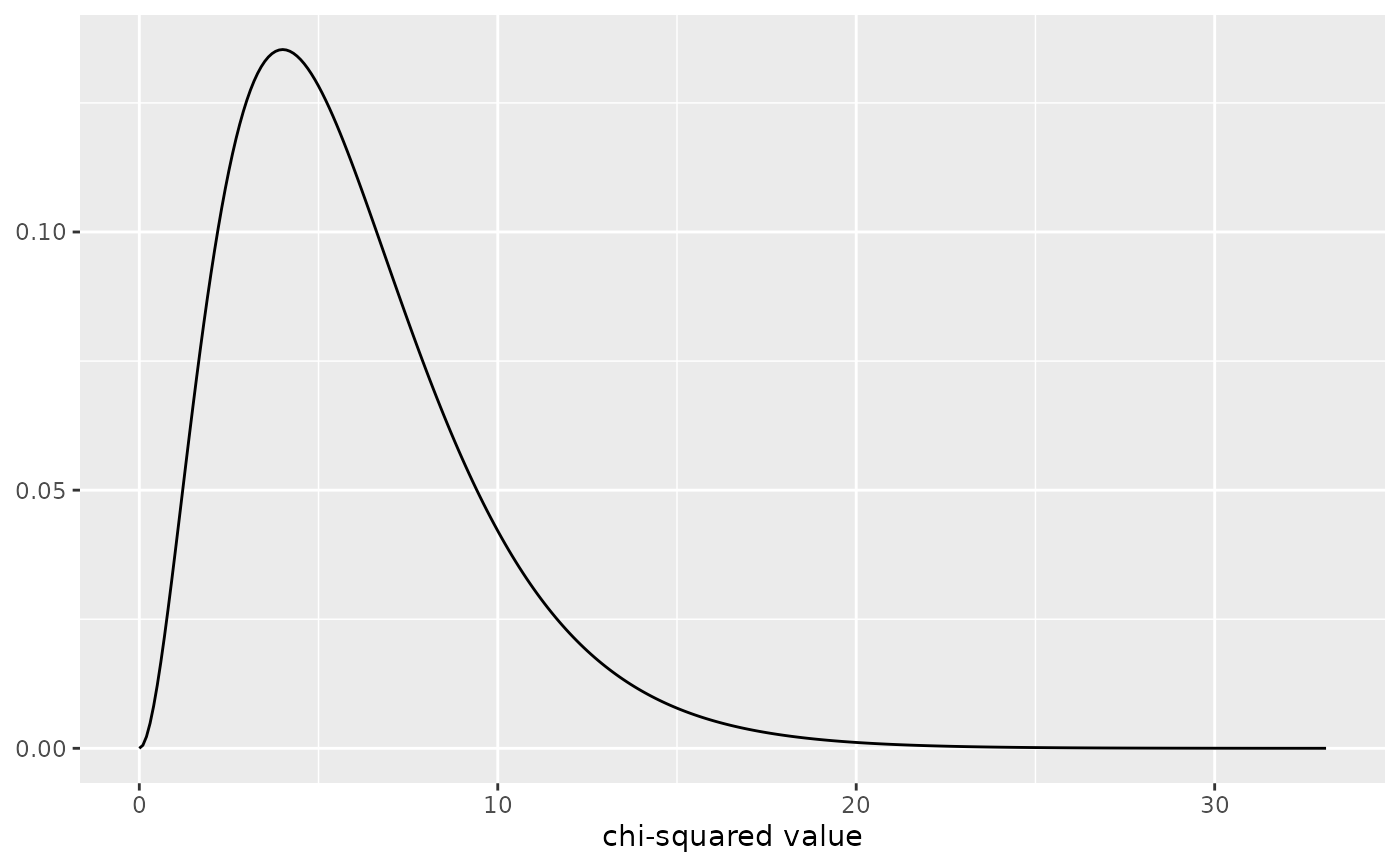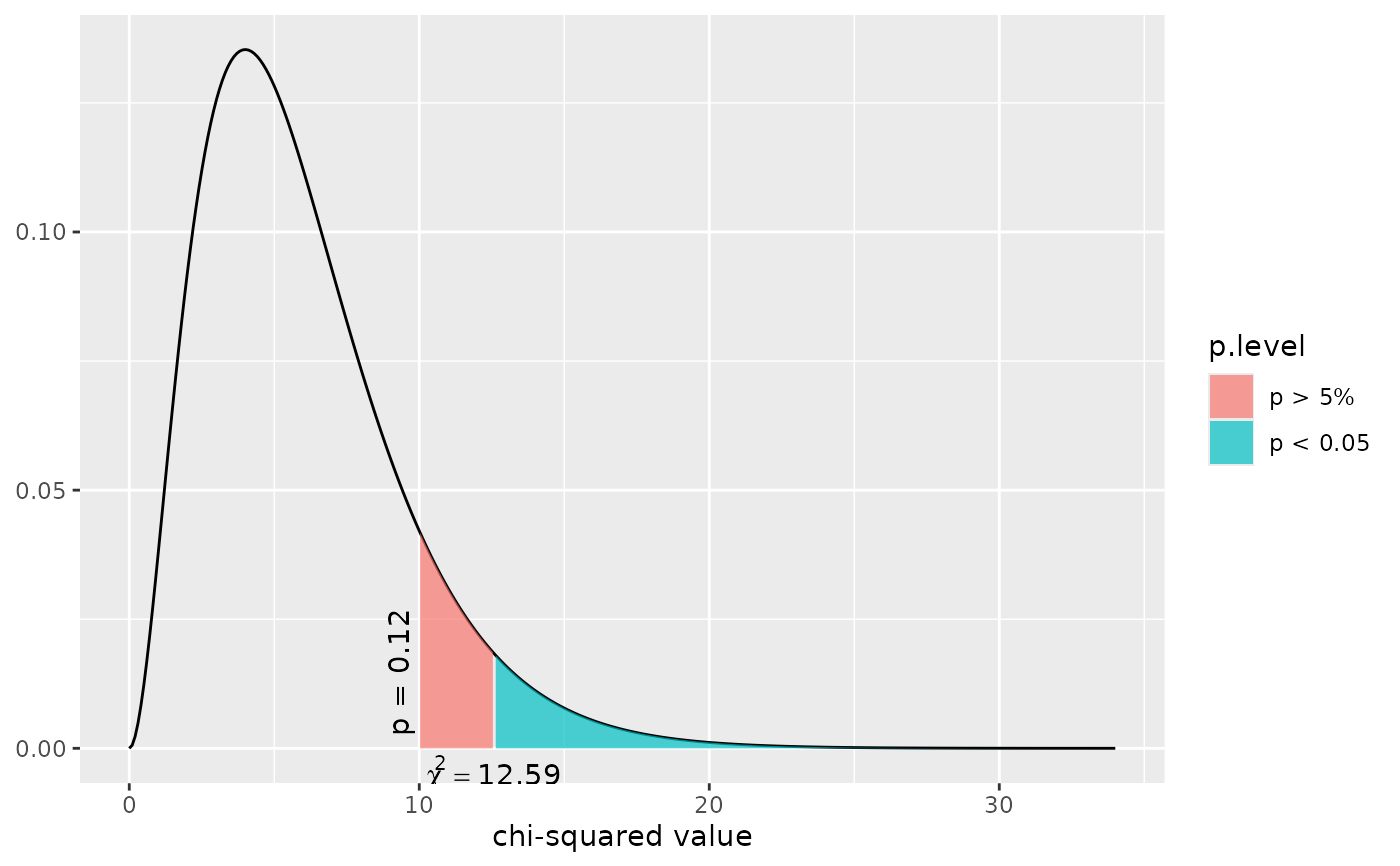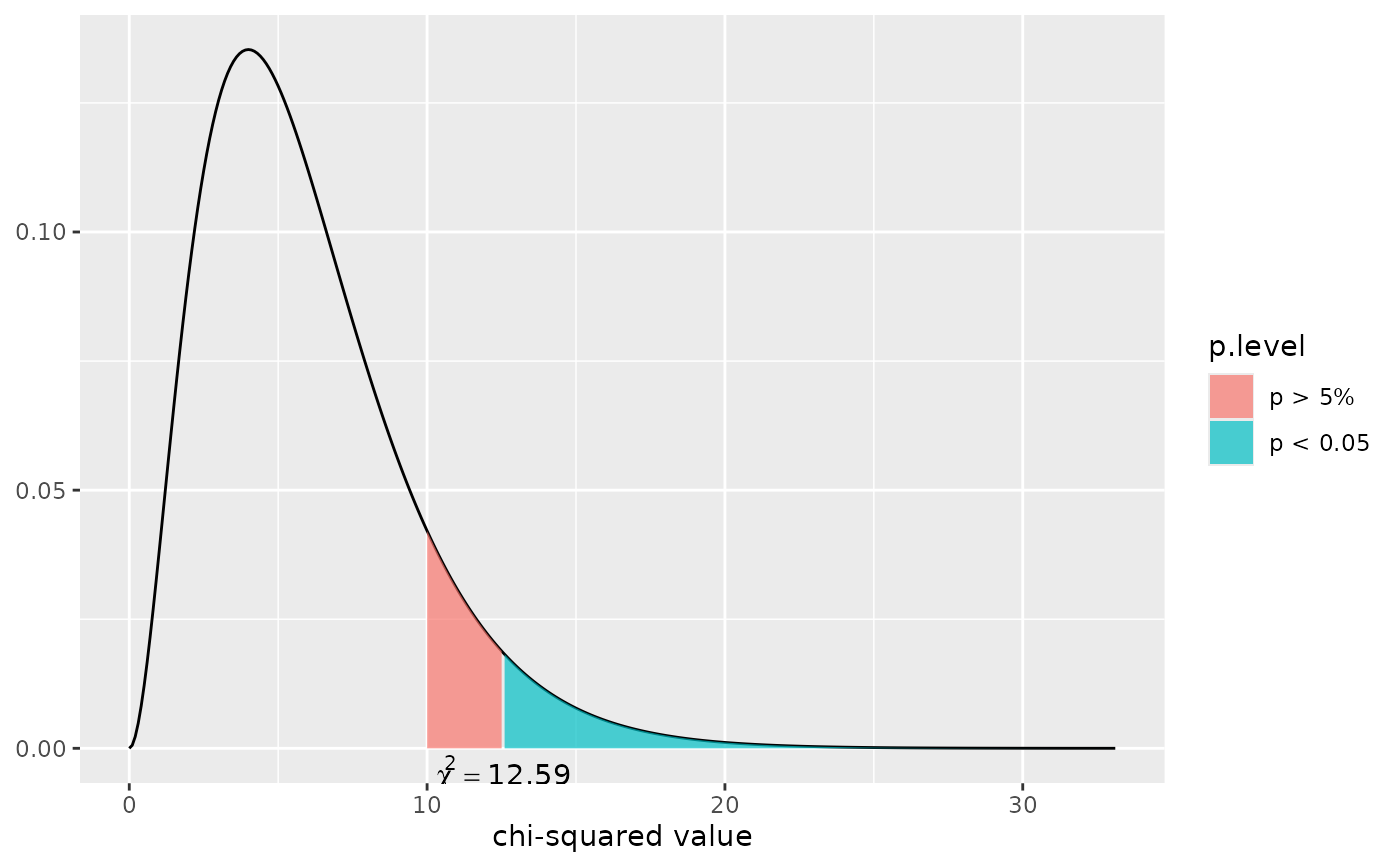This function plots a simple chi-squared distribution or a chi-squared distribution with shaded areas that indicate at which chi-squared value a significant p-level is reached.

dist_chisq(
chi2 = NULL,
deg.f = NULL,
p = NULL,
xmax = NULL,
geom.colors = NULL,
geom.alpha = 0.7
)

Arguments

chi2

Numeric, optional. If specified, a chi-squared distribution with deg.f degrees of freedom is plotted and a shaded area at chi2 value position is plotted that indicates whether or not the specified value is significant or not. If both chi2 and p are not specified, a distribution without shaded area is plotted.

deg.f

Numeric. The degrees of freedom for the chi-squared distribution. Needs to be specified.

p

Numeric, optional. If specified, a chi-squared distribution with deg.f degrees of freedom is plotted and a shaded area at the position where the specified p-level starts is plotted. If both chi2 and p are not specified, a distribution without shaded area is plotted.

xmax

Numeric, optional. Specifies the maximum x-axis-value. If not specified, the x-axis ranges to a value where a p-level of 0.00001 is reached.

geom.colors

user defined color for geoms. See 'Details' in plot_grpfrq.

geom.alpha

Specifies the alpha-level of the shaded area. Default is 0.7, range between 0 to 1.

Examples

# a simple chi-squared distribution
# for 6 degrees of freedom
dist_chisq(deg.f = 6)# a chi-squared distribution for 6 degrees of freedom,
# and a shaded area starting at chi-squared value of ten.
# With a df of 6, a chi-squared value of 12.59 would be "significant",
# thus the shaded area from 10 to 12.58 is filled as "non-significant",
# while the area starting from chi-squared value 12.59 is filled as
# "significant"
dist_chisq(chi2 = 10, deg.f = 6)# a chi-squared distribution for 6 degrees of freedom,
# and a shaded area starting at that chi-squared value, which has
# a p-level of about 0.125 (which equals a chi-squared value of about 10).
# With a df of 6, a chi-squared value of 12.59 would be "significant",
# thus the shaded area from 10 to 12.58 (p-level 0.125 to p-level 0.05)
# is filled as "non-significant", while the area starting from chi-squared
# value 12.59 (p-level < 0.05) is filled as "significant".
dist_chisq(p = 0.125, deg.f = 6)